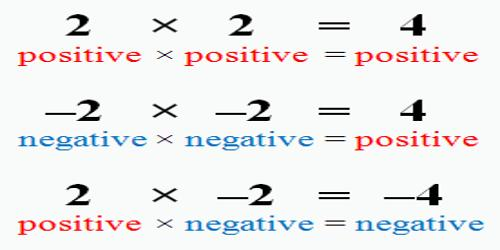Multiplication of Integers: Negative and Positive

Multiplication of Integers is similar to multiplication of whole numbers (both positive) except the sign of the product needs to be determined.

RULE 1: The product of a positive integer and a negative integer is negative.

RULE 2: The product of two positive integers is positive.

RULE 3: The product of two negative integers is positive.

Examples:

Rule 1: 1. (+4) x (-2) = -8        2. (-2) x (+5) = -10

Rule 2: 1. (+6) x (+8) = +48    2. (+6) x (+2) = +12

Rule 3: 1. (-6) x (-8) = +48      2. (-2) x (-4) = +8

In other words, if the signs are the same the product will be positive, if they are different, the product will be negative.Explanation

Multiplying positive and negative numbers has far less rules than adding or subtracting positive and negative numbers, in fact there are only three that you’ll have to remember:

Rule 1: A positive number times a positive number gives you a positive number.

Example 1: This is the kind of multiplication you’ve been doing for years, positive numbers times positive numbers. It would look like this: 4 x 3 = 12. 4 is positive, 3 is positive, thus, 12 is positive. We know that 4 and 3 are both positive because there are no negative signs in front of them.

Rule 2: A positive number times a negative number gives you a negative number.

Example 2: This is new – for example, you might have 4 x -3. The 4 is positive, but the 3 is negative, so our answer has to be negative. Thus, we multiply the numbers together as we normally would, and then put a negative sign in front of our answer. So, 4 x -3 = -12. Please note that this also works when the negative number comes first and the positive number is second. For example, you may see it written -3 x 4, but don’t get confused. The combination of one positive and one negative number, no matter which order they come in, means your answer is going to be negative.

Rule 3: A negative number times a negative number gives you a positive number.

Example 3: This is also new—and doesn’t seem to make much sense, but it is a rule we have to follow when multiplying negative numbers together. So, for example, we may have the problem -3 x -4. Both the 3 and the 4 are negative, so we know our answer is going to be positive. Therefore, -3 x -4 = 12.

Information Source: